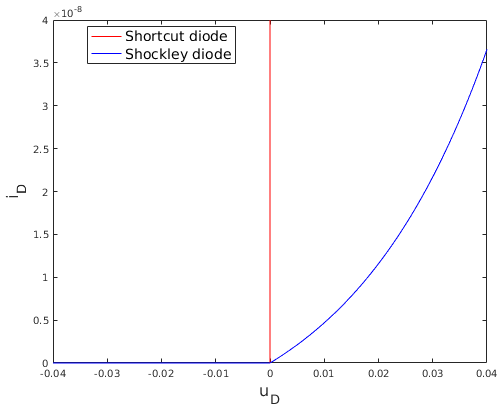### RLC circuit with diode

• Shortcut diode:
• all components including Diode in MSL
• implementation uses standard "s trick"
• ```off = s < 0;
v = if off then s else 0;
i = if off then 0 else s;```
• variable s is curve parameter along characteristic
• Shockley diode:
• easily implemented using a partial model from MSL
• piecewise linear interpolated approximation
• mainly graphically with LookupTable and VoltageSensor from MSL
• “Real-time” variant:
• replace characteristic equation by its derivative
• → reduces DAE to ODE (at least for simplified total model)
• here: i = 0 remains in diode component
• diode switches from locking to conducting phase
• → i changes from algebraic to state variable
• works in MapleSim, not in Dymola
• workaround: use der(i) = 0 instead